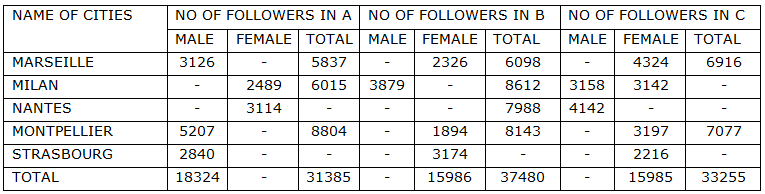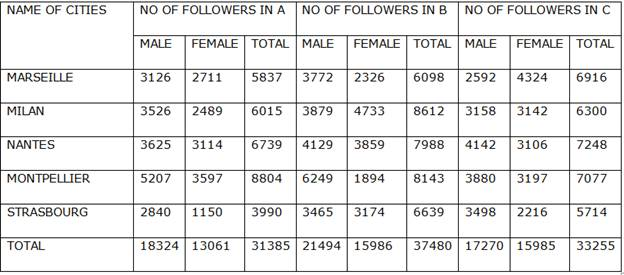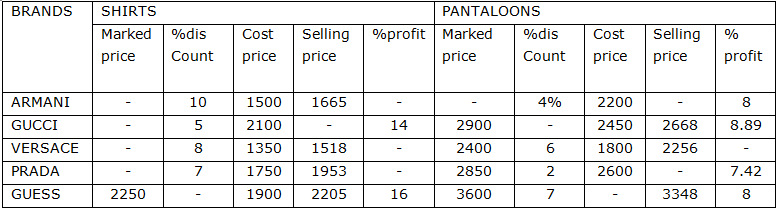Q.81-85) Observes the following table and answer the questions accordingly.

The following table gives the detail about the no of followers for three different rock bands A, B and C in different cities.Q.1) What is the no of female followers for rock band B in Milan city?

a) 4422

b) 4183

c) 3991

d) 4733

e) None of these

d

The no of followers for rock band B in Milan city is 4733

Q.2) What is the respective ratio of the no of male followers for rock band A in Strasbourg to the no of male followers for rock band C in Montpellier?

a) 83:31

b) 67:79

c) 71:97

d) 56:87

e) None of these

Required ratio=2840:3880=71:97

Q.3) What is the difference between the no of male followers for rock band B in all the cities and the no of female followers for rock band A in all the cities?

a) 7988

b) 6354

c) 8433

d) 9112

e) None of these

Required difference=21494-13061=8433

Q.4) The no of female followers in Nantes for all rock bands is what percent of the no of total female followers?

a) 27%

b) 22%

c) 29%

d) 25%

e) None of these

b

The no of female followers in Nantes for all rock bands=3114+3859+3106=10079

Total no of females in all cities=13061+15986+15985=45032

Required percentage =10079/45032×100=22.38%

Q.5) What is the average no of male followers are there for all the rock band in all the cities?

a) 19029

b) 10929

c) 19092

d) 19209

e) 10992

a

Average no of male followers in all cities for all the rock bands =(18324+21494+17270)/3

=57088/3=19029

Q.6-10) Observe the following table and answer the questions accordingly.

The following table shows the details about the sale of outfits of different brands at different costs.Q.6) What is the cost price of 11 GUESS branded pantaloons in rs.?

a) 34100

b) 31150

c) 32550

d) 33690

e) None of these

a

The % profit in selling a pantaloon =3348/108×100=3100

The cost price of 11 pantaloons =11×3100=34100rs.

Q.7) Which of the following product gives out the maximum percentage of profit in selling and how much is the amount?

a) Versace pantaloon, 456rs.

c) Guess shirt, 305rs.

d) Gucci pantaloon, 216rs.

e) None of these

a

From the table, we can conclude that selling a Versace pantaloon gives out the maximum percentage of profit and it is 456rs.

Q.8) If three Armani pantaloons and four shirts of same brand is bought a person will get 4% off on shirts and 2% off on pantaloons. What will be the total cost of those items in rs.?

a) 13779

b) 13379

c) 13977

d) 13397

e) None of these

b

Cost price of 4 shirts=1665×4=6660rs.

If 4% offer is given, Selling price=6660/100×96=6393.6rs.

Cost price of 3 pantaloons= 2376×3=7128rs.

If 2% offer is given, Selling price=7128/100×98=6985.4rs.

Total cost=6393.6+6985.4=13379rs.

Q.9) What is the difference between selling 3 Armani pantaloons and 4 Gucci shirts if no offer is given for both the brands in rs.?

a) 2890

b) 2770

c) 2550

d) 2655

e) None of these

d

If no offer is given, then the selling price would be the marked price.

Marked price of Armani pantaloons =2376/96×100=2475rs.

Marked price of Gucci shirts =2394/95×100=2520rs.

Required difference=(2475×3)-(2520×4)=7425-10080=2655rs.

Q.10) If a customer demands an extra 2.5% discount on the marked price on buying a Gucci pantaloon what will be the overall profit or loss percentage for the seller?

a) 10.3% loss

b) 9.5% loss

c) 6.1% profit

d) 8.1% profit

e) 7.8% profit

c

The marked price of pantaloon=2900rs.

Selling price=2668rs.

Discount=232rs.

Discount price =232/2900×100=8%

The overall discount on marked price = -8-2.5+(-8×-2.5)/100=-10.3%

So, the selling price would be,

=2900-2900/100×10.3=2900-298.7=2601.3rs.

Cost price=2450 rs.

Profit=2601.3-2450=151.3rs

Required percentage =151.3/2450×100=6.17% profit